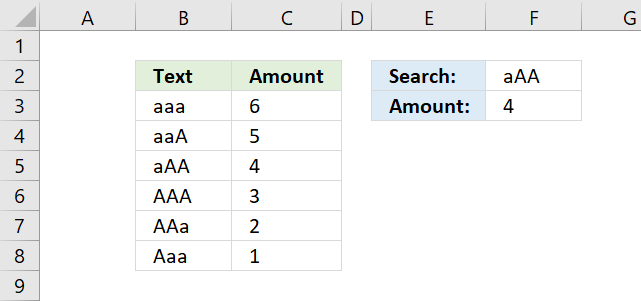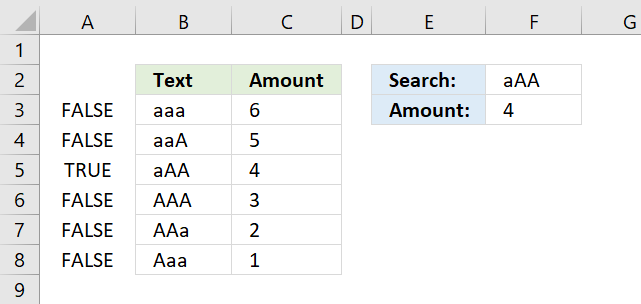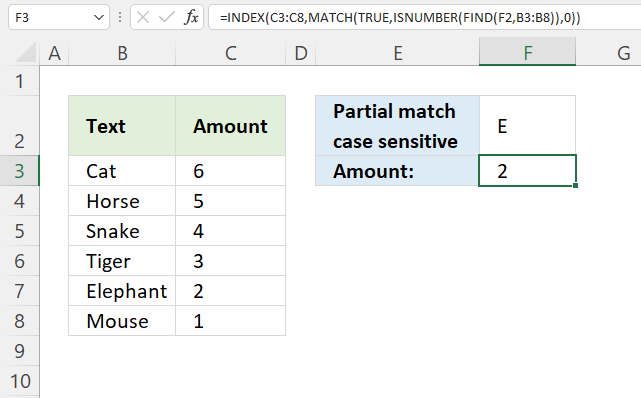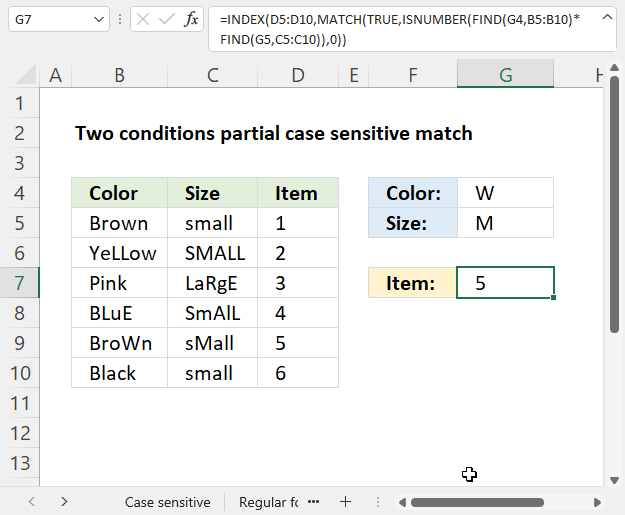Author: Oscar Cronquist Article last updated on November 16, 2021The picture above demonstrates a formula in cell F3 that allows you to look up a value in column B using the value in cell F2, also considering letter casing, then return the corresponding value from column C.

## 1. How to do a case sensitive INDEXMATCH=INDEX(C3:C8,MATCH(TRUE,EXACT(F2,B3:B8),0))

### 1.1 How to enter an array formula

The formula above is an array formula. To enter an array formula, type the formula in a cell then press and hold CTRL + SHIFT simultaneously, now press Enter once. Release all keys.

The formula bar now shows the formula enclosed with curly brackets telling you that you entered the formula successfully. Don't enter the curly brackets yourself.

If you prefer a regular formula, read "Alternative regular formula" below in this article.

### 1.2 Explaining formula in cell F3

#### Step 1 - Compare lookup value with lookup column

The EXACT function allows you to compare values, if they are exactly the same the EXACT function returns TRUE. Note, the function is case-sensitive.

EXACT(F2,B3:B8)

becomes

EXACT("aAA",{"aaa"; "aaA"; "aAA"; "AAA"; "AAa"; "Aaa"})

and returns

{FALSE; FALSE; TRUE; FALSE; FALSE; FALSE}.#### Step 2 - Identify the relative position of value TRUE in the array

The MATCH function finds a specific value in an array or cell range and returns its location, a number representing the position.

MATCH(TRUE,EXACT(F2,B3:B8),0)

becomes

MATCH(TRUE, {FALSE; FALSE; TRUE; FALSE; FALSE; FALSE}, 0)

and returns 3. TRUE is in the third position in the array.

#### Step 3 - Return corresponding value from column C

The INDEX function returns a value from an array or cell range based on the location. That is why the INDEX and MATCH functions work so well together.

INDEX(C3:C8,MATCH(TRUE,EXACT(F2,B3:B8),0))

becomes

INDEX(C3:C8,3)

becomes

INDEX({6;5;4;3;2;1},3)

and returns 4 in cell F3.

## 2. Alternative regular formulaFormula in cell F2:

=INDEX(C3:C8, MATCH(TRUE, INDEX(EXACT(F2, B3:B8), ), 0))

## 3. How to do a case sensitive partial match using INDEX and MATCH functionsFormula in cell F3:

=INDEX(C3:C8, MATCH(TRUE, ISNUMBER(FIND(F2, B3:B8)), 0))

### 3.1 Explaining formula in cell F3

#### Step 1 - Perform a case sensitive search (partial match)

The FIND function returns a number representing the position of a specific substring in another string, reading left to right. Note, the FIND function is case-sensitive.

FIND(F2, B3:B8)

becomes

FIND("E", {"Cat"; "Horse"; "Snake"; "Tiger"; "Elephant"; "Mouse"})

and returns

{#VALUE!; #VALUE!; #VALUE!; #VALUE!; 1; #VALUE!}

Notice the error values in the array above, they appear if the substring is not found for each value in cell range B3:B8.

#### Step 2 - Identify numbers

The ISNUMBER function checks if a value is a number, it returns either TRUE or FALSE.

ISNUMBER(FIND(F2, B3:B8))

becomes

ISNUMBER({#VALUE!; #VALUE!; #VALUE!; #VALUE!; 1; #VALUE!})

and returns

{FALSE; FALSE; FALSE; FALSE; TRUE; FALSE}.

#### Step 3 - Find the position of the first number in the array

The MATCH function returns a number representing the relative position of an item in an array or cell reference that matches a specified value in a specific order.

MATCH(lookup_value, lookup_array, [match_type])

MATCH(TRUE, ISNUMBER(FIND(F2, B3:B8)), 0)

becomes

MATCH(TRUE, {FALSE; FALSE; FALSE; FALSE; TRUE; FALSE}, 0)

and returns 5. TRUE is first found in position five in the array above.

#### Step 4 - Get corresponding value from the same row

The INDEX function returns a value from a given cell range based on a row and column number (optional).

INDEX(array, [row_num], [column_num], [area_num])

INDEX(C3:C8, MATCH(TRUE, ISNUMBER(FIND(F2, B3:B8)), 0))

becomes

INDEX(C3:C8, 5)

becomes

INDEX({6;5;4;3;2;1}, 5)

and returns 2.

## 4. How to do a partial case sensitive match using two conditions in two columnsFormula in cell F3:

=INDEX(D5:D10, MATCH(TRUE, ISNUMBER(FIND(G4, B5:B10)*FIND(G5, C5:C10)), 0))

### 4.1 Explaining formula in cell F3

#### Step 1 - Perform a case sensitive search (partial match)

The FIND function returns a number representing the position of a specific substring in another string, reading left to right. Note, the FIND function is case-sensitive.

FIND(find_text,within_text, [start_num])

FIND(G4, B5:B10)

becomes

FIND("W", {"Brown"; "YeLLow"; "Pink"; "BLuE"; "BroWn"; "Black"})

and returns

{#VALUE!; #VALUE!; #VALUE!; #VALUE!; 4; #VALUE!}.

#### Step 2 - Perform a second case sensitive search (partial match)

FIND(G5, C5:C10)

becomes

FIND("M",{"small";"SMALL";"LaRgE";"SmAlL";"sMall";"small"})

and returns

{#VALUE!; 2; #VALUE!; #VALUE!; 2; #VALUE!}.

#### Step 3 - Multiply arrays

The formula returns a value if both substrings are found on the same row, to do that we need to apply AND logic.

The asterisk character lets you multiply values row by row, if both values are numbers the result is a number.

#VALUE! * #VALUE! = #VALUE!
#VALUE! * 2 = #VALUE!
2 * #VALUE! = #VALUE!
2*2 = 4

FIND(G4, B5:B10)*FIND(G5, C5:C10)

becomes

{#VALUE!; #VALUE!; #VALUE!; #VALUE!; 4; #VALUE!} * {#VALUE!; 2; #VALUE!; #VALUE!; 2; #VALUE!}

and returns

{#VALUE!; #VALUE!; #VALUE!; #VALUE!; 8; #VALUE!}.

#### Step 4 - Identify numbers

The ISNUMBER function checks if a value is a number, it returns either TRUE or FALSE.

ISNUMBER(FIND(G4, B5:B10)*FIND(G5, C5:C10))

becomes

ISNUMBER({#VALUE!; #VALUE!; #VALUE!; #VALUE!; 8; #VALUE!})

and returns {FALSE; FALSE; FALSE; FALSE; TRUE; FALSE}.

#### Step 5 - Find the position of the first number in the array

The MATCH function returns a number representing the relative position of an item in an array or cell reference that matches a specified value in a specific order.

MATCH(lookup_value, lookup_array, [match_type])

MATCH(TRUE, ISNUMBER(FIND(G4, B5:B10)*FIND(G5, C5:C10)), 0)

becomes

MATCH(TRUE, {FALSE; FALSE; FALSE; FALSE; TRUE; FALSE}, 0)

and returns 5.

#### Step 6 - Get corresponding value on the same row

The INDEX function returns a value from a given cell range based on a row and column number (optional).

INDEX(array, [row_num], [column_num], [area_num])

INDEX(D5:D10, MATCH(TRUE, ISNUMBER(FIND(G4, B5:B10)*FIND(G5, C5:C10)), 0))

becomes

INDEX(D5:D10, 5)

becomes

INDEX({1;2;3;4;5;6}, 5)

and returns 5.